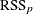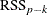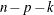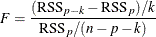#### Backward Elimination (BACKWARD)

The backward elimination technique starts from the full model including all independent effects. Then effects are deleted one by one until a stopping condition is satisfied. At each step, the effect showing the smallest contribution to the model is deleted. In traditional implementations of backward elimination, the contribution of an effect to the model is assessed by using an F statistic. At any step, the predictor producing the least significant F statistic is dropped and the process continues until all effects remaining in the model have F statistics significant at a stay significance level (SLS).

More precisely, if the current model has p parameters excluding the intercept, and if you denote its residual sum of squares byand you drop an effect with k degrees of freedom and denote the residual sum of squares of the resulting model by, then the F statistic for removal with k numerator degrees of freedom anddenominator degrees of freedom is given bywhere n is number of observations used in the analysis.

Just as with forward selection, you can change the criterion used to assess effect contributions with the SELECT= option. You can also specify a stopping criterion with the STOP= option and use a CHOOSE= option to provide a criterion used to select among the sequence of models produced. See the discussion in the section Forward Selection (FORWARD) for additional details.

##### Examples of Backward Selection Specifications
selection=backward


removes effects that at each step produce the largest value of the Schwarz Bayesian information criterion (SBC) statistic and stops at the step where removing any effect increases the SBC statistic.

selection=backward(stop=press)


removes effects based on the SBC statistic and stops at the step where removing any effect increases the predicted residual sum of squares (PRESS).

selection=backward(select=SL)


removes effects based on significance level and stops when all candidate effects for removal at a step have a significance level less than the default stay significance level of 0.15.

selection=backward(select=SL choose=validate SLS=0.1)


removes effects based on significance level and stops when all effects in the model are significant at the 0.1 level. Finally, from the sequence of models generated, choose the one that gives the smallest average square error when scored on the validation data.